# GMAT Shortcut: Adding to the Numerator and Denominator

First, try these practice DS questions:

1) If x and y are positive integers, is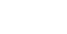?

Statement #1: y > 20
Statement #2: x < 5

2) If x and y are positive integers, is?

Statement #1: y = 5
Statement #2: x > y

Throughout this post, assume that I am talking about positive fractions with a positive numerator and positive denominator.  If the fraction is negative, use the information below to figure out what happens to the absolute value of the fraction, and judge from there.

Ironically, it’s a bit easier if we add to part of the fraction and subtract from the other.

The BIG idea here: if you increase the numerator and/or decrease the denominator of any positive fraction, that fraction will get bigger; if you decrease the numerator and/or increase the denominator of any positive fraction, that fraction will get smaller.

Add a positive number to the numerator and/or subtract a positive number from the denominator of any positive fraction, and the new fraction will be greater than the starting fraction.  Subtract a positive number from the numerator and/or add a positive number to the denominator of any positive fraction, and the new fraction will be smaler than the starting fraction.  Though not relevant in the two practice problems above, this is a golden rule that will help you in a panoply of fraction and ratio problems.

## Adding the Same Number to Numerator and Denominator

Suppose we start with the positive fraction x/y and we want to add some positive number b to both the numerator and the denominator.  How does the resultant fraction, (x + b)/(y + b), compare to the starting fraction?

Well, the rule here is a bit subtle.  When you add the same number to numerator and denominator, the resultant fraction is closer to 1 than is the starting fraction.  This means, if the starting fraction x/y is less than 1, then the resultant fraction is closer to one — bigger than the starting fraction.  If the starting fraction x/y is an “improper fraction”, a fraction with a value greater than one, than adding the same number to both the numerator and the denominator will make the resultant fraction closer to 1 — less than the starting fraction.

Here are a couple of examples.

Example #1

Start = 2/3 —- a fraction less than one.

Add five to the numerator and the denominator.

Result = 7/8 — this fraction is closer to one than is 2/3: on the number line —–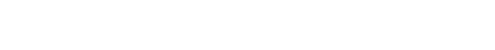Since 1 is bigger than 2/3, when the resultant fraction moved closer to 1, it got bigger than 2/3.  Therefore, we know 2/3 < 7/8

Example #2

Start = 3/2 —- a fraction greater than one.

Add two to the numerator and the denominator.

Result = 5/4 — this fraction is closer to 1 than is 3/2: on the number line —–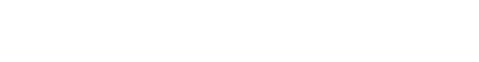Since 1 is less than 3/2, when the result fraction moved closer to 1, it got smaller than 3/2.  Therefore, we know 3/2 > 5/4

## Adding Different Numbers to the Numerator and Denominator

Actually, this case is simply a generalization of the previous case.  Suppose we start with a fraction x/y, and we add the positive number a to the numerator and the positive number b to the denominator, and we want to know if the resultant fraction is bigger or smaller than the starting fraction.

Well, the general rule is: adding a to the numerator and b to the denominator moves the resultant fraction closer to the fraction a/b.  If x/y < a/b, moving the starting fraction close to a/b will make it bigger.  If x/y > a/b, moving the starting fraction close to a/b will make it smaller.

Here are some example:

Example #3

Start = 2/7

Add 3 to the numerator and 5 to the denominator.

Resultant fraction = 5/12— this fraction is closer to 3/5 than is 2/7 —-

On the number line —–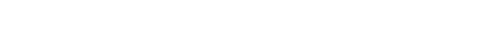Because 3/5 is bigger than 2/7, adding 3 to the numerator and 5 to the denominator has the net effect of producing a fraction that is bigger: 2/7 < 5/12

Example #4

Start = 11/12

Add 2 to the numerator and 5 to the denominator.

Resultant fraction = 13/17— this fraction is closer to 2/5 than is 11/12 —-

On the number line —–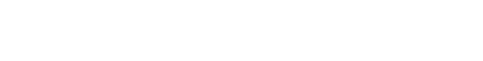Because 2/5 is less than11/12, adding 2 to the numerator and 5 to the denominator has the net effect of producing a fraction that is smaller: 11/12 > 13/17

Now that you know these rules, go back to the practice problems at the beginning and see whether they make more sense now.

## Practice Problem Solutions

1) Statement #1: We are adding 2 to the numerator and 3 to the denominator, so we know the resultant fraction will move closer to 2/3.  If all we know is that the denominator of the starting fraction is greater than 20, then we have no idea what the size of the starting fraction is: it could be much greater than 2/3, or much smaller than 2/3, depending on the numerator, of which we have no idea.  We can draw no conclusion right now.  This statement, alone, by itself, is insufficient.

Statement #2: Now, all we know is that the numerator of the starting fraction is less than 5 — it could be 4, 3, 2, or 1.  We have no idea of the denominator.  If y = 50, then we get a very small fraction.  But if x = 4 and y = 1, the fraction equals 4, much larger than 2/3.  In this statement, we have no information about the denominator, and since we know nothing about the denominator, we know nothing about the size of the starting fraction: it could be either greater or less than 2/3.  Therefore, we can draw no conclusion.  This statement, alone, by itself, is also insufficient.

Now, combine the statements.  We know y > 20 and x < 5.  Well no matter what values we choose, we are going to have a denominator much bigger than the numerator.  The larger possible fraction we could have under these constraints would be 4/21 (largest possible numerator with smallest possible denominator).  The fraction 4/21 is much smaller than 1/2, so it’s definitely smaller than 2/3.  Any fraction with y > 20 and x < 5 will be less than 2/3.  Therefore, adding 2 to the numerator and 3 to the denominator will move the resultant fraction closer to 2/3, which has the net effect of increasing its value.  Therefore, the answer to the prompt question is “yes.”  Because we can give a definite answer to the prompt, we have sufficient information.

Neither statement is sufficient individually, but together, they are sufficient.  Answer = C.

2) We are adding the same number, 5, to both the numerator and the denominator, so the value of x/y will move closer to 1.  All we need to determine is whether x/y is greater than 1 or less than 1.

Statement #1: y = 5.  Here, we have a definite value for y, but zero information about x.  If y = 5, some fractions (1/5) can be less than one, while others (7/5) will be greater than one.  Either is possible.  Since both are possible, we can’t give a definitive answer to the prompt.  This statement, alone, by itself, is insufficient.

Statement #2: x>y.  Dividing both sides of this inequality by y, we get (x/y) > 1.  This means x/y must be a fraction greater than 1, which means the resultant fraction (x + 5)/(y + 5) must be closer to one, which means the resultant fraction must be smaller.  Therefore, we can definitively say: the answer to the prompt question is, “No.”  Because we can give a definite answer to the prompt, we have sufficient information.  This statement, alone, by itself, is sufficient.

Statement #1 is insufficient and Statement #2 is sufficient.  Answer = B.

## Author

•Mike served as a GMAT Expert at Magoosh, helping create hundreds of lesson videos and practice questions to help guide GMAT students to success. He was also featured as "member of the month" for over two years at GMAT Club. Mike holds an A.B. in Physics (graduating magna cum laude) and an M.T.S. in Religions of the World, both from Harvard. Beyond standardized testing, Mike has over 20 years of both private and public high school teaching experience specializing in math and physics. In his free time, Mike likes smashing foosballs into orbit, and despite having no obvious cranial deficiency, he insists on rooting for the NY Mets. Learn more about the GMAT through Mike's Youtube video explanations and resources like What is a Good GMAT Score? and the GMAT Diagnostic Test.